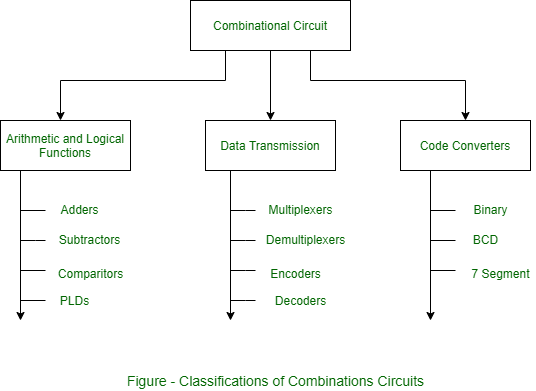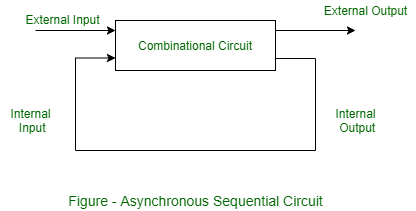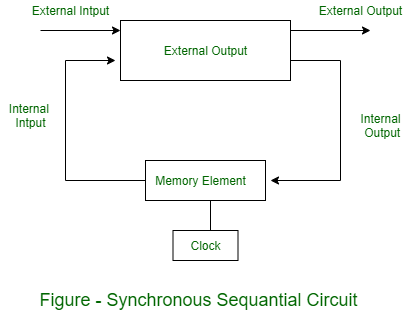# Classifications of Combinational and Sequential circuits

1. Classifications of Combinational Circuits:
There are three main categories of combinational circuits: arithmetic or logical functions, data transmission and code converter as given below in category diagram.Functions of Combinational circuits are generally expressed by Boolean algebra, Truth table, or Logic diagram. The Boolean algebra is a logic function that is expressed in the form of expression. The Truth table contains outputs of all possible inputs. Whereas Logic diagram is a graphical representation of logic functions using logic gates. The diagram of a combinational circuit has logic gates with no feedback paths or memory elements. All the three representations are always equal for a specific logic circuit.

2. Sequential circuits:
Now, these are types and classifications of Sequential circuits.

Types of Sequential circuits:
The sequential circuits can be event driven, clock driven and pulse driven. There are two main types of sequential circuits: (a) Synchronous and (b) Asynchronous.

• (a). Asynchronous Sequential circuits
Asynchronous circuits do not synchronize with positive edge or negative edge of the clock signal, that means, the outputs of asynchronous sequential circuits do not change or affect at the same time and change their state immediately when there is a change in the input signal. So, these circuits are faster and independent of the internal clock pulses. But these circuits have uncertainty in the outputs and are difficult to design.• (b). Synchronous Sequential circuits
Synchronous circuits synchronize with either positive edge or negative edge of the clock signal, that means, the outputs of synchronous sequential circuits change or affect at the same time. These circuits use clock signal and level input (or pulsed with restrictions on pulse width and circuit propagation). Since they wait for the next clock pulse to arrive to perform the next operation, so these circuits are a bit slower compared to asynchronous. Level output changes state at the start of an input pulse and remains in that until the next input or clock pulse. The synchronous sequential circuit can be locked or unlocked (or pulsed).Counters, Flip-Flops, and design of Mealy-Moore machines are examples of Synchronous sequential circuits.

Attention reader! Don’t stop learning now. Get hold of all the important CS Theory concepts for SDE interviews with the CS Theory Course at a student-friendly price and become industry ready.

My Personal Notes arrow_drop_upIf you like GeeksforGeeks and would like to contribute, you can also write an article using contribute.geeksforgeeks.org or mail your article to contribute@geeksforgeeks.org. See your article appearing on the GeeksforGeeks main page and help other Geeks.

Please Improve this article if you find anything incorrect by clicking on the "Improve Article" button below.